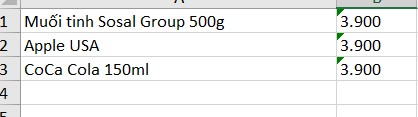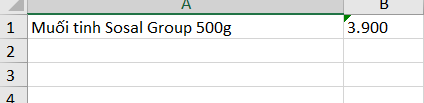# Extract the multi row value in excel file

I have a file iinput excel contain 1 column. I have to use the value of column to search on the web page and extract the data ( contain 2 column).But I just can extract 1 row of data instead of multi row.
Can anyone healp me plz? I use REFramework for the problem.

can you share any sample?

Hi @Le_Vo_Tien_Phat_KHCM - Can you share the screenshot or some dummy input file

Hi @ushu, @Manzar.Ali ,

Here is the input example. With each row of the input I will use the value to type into on the web site to buy goods. Next , I will extract the smallest price item of all the goods in webpage. Lastly, I have to write the price and the name of the item above in the new excel file. But I just can extract 1 row of data instead of multi row? Thanks for helpHI @Manzar.Ali @ushu ,
If i say something you are not understading , may I share the file zip project for you guy. And you can see the problem clearlier

@Le_Vo_Tien_Phat_KHCM - It sounds like you want to extract the multiple rows from a web page. Did you try with data scraping

Studio - About Data Scraping (uipath.com)

Hi @ushu ,
No bro, The step scraping the data i have passed. The next step is I will choose the smallest value of these of data I scrap before and extract them in a new file excel

@Le_Vo_Tien_Phat_KHCM - You want to find the samllest(min) value from the data table that you scrape. If so, please check below one

Find Max, Min, Average value from a DataTable UiPath - RPA Learners

As per your response, you have completed till the scraping process. So, the scraped data will be stored in the form of datatable type.

1. To find the minimum value - Use assign activity
minimumValue = dt.AsEnumerable.Min(Function(row) Cint(row(“ColumnName”)))
2. To find the maximum value - Use assign activity
maximumValue = dt.AsEnumerable.Max(Function(row) Cint(row(“ColumnName”)))

Note:

1. dt - represents the datatable variable.
2. minimumValue & maximumValue are two variables which needs to initialize with default value as 0

To write the value into an excel file, you can use Add Data Row, pass the values and finally Write Range.

Thanks,
@90s_Developer

Exactly the problem i am facing is in writing the final result in excel file.
What i need is file have multi rows like this1. Initialize an empty datatable variable resultDt & empty datarow variable resultDtrow
2. Use Read Range activity to read the respective excel from the local folder (outputDt)
3. Use Assign activity with the below expression.
outputDt = (From row In outputDt.AsEnumerable() Order By Double.Parse(row.Field(Of String)(“ColumnName”)) Ascending Select row).CopyToDatatable()

This will sort the datatable based on the Column B

1. Use Assign activity to clone the input datatable with empty rows.
resultDt = outputDt.Clone

2. Use Assign activity,
resultDtrow = resultDt .Rows.Add((From row In outputDt.AsEnumerable() Select row).First().ItemArray)

This will retrieve the first row which contains minimum value

1. Use Assign activity,

This will retrieve the first row which contains maximum value

1. Use Write Range activity and pass the resultDt variable.

Now, you will have the rows which has minimum value and maximum rows.

Note: If you require only minimum value of the datatable, you can skip the step no: 5 & 6

And replace this step before you perform step 7
Use Assign activity,

resultdt = (From row In outputDt.AsEnumerable() Select row).Take(1).CopyToDataTable().Clone()